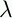September 22, 1999 Perl and the Lambda Calculus Slide #35

# There's a Catch

• Perl uses applicative order evaluation for functions

• If it works for Perl, why not for-calculus?

• Answer: It doesn't always work for Perl

• Example: You can't write a function that behaves like if:

```        sub my_if {
my (\$cond, \$then, \$else) = @_;
if (\$cond) { return \$then }
else       { return \$else }
}
```
```        sub fact {
my \$n = shift;
return my_if(\$n == 0, 1, \$n*fact(\$n-1));
}
```
• Infinite loop on all inputs

• If we use Perl functions as-expressions, we always get applicative-order evaluation

• For IF and Y, this doesn't work

• We can't change Perl's evalutation order

 NextCopyright © 1999 M-J. Dominus